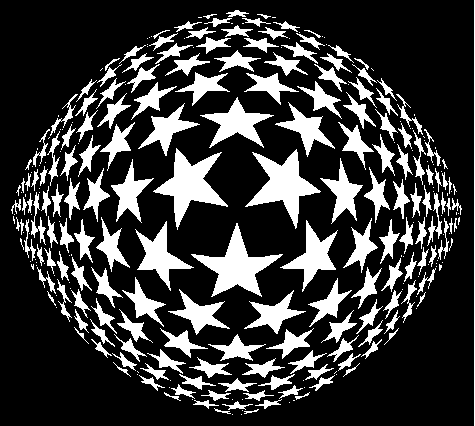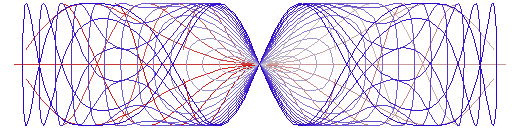# Geometry: Transformation of the Plane II

By Xah Lee. Date: . Last updated: .

The following are images of transformations in the plane.Saturn. The preimage and image of a linear transformation on a polar grid by the matrix {{3,-2},{1,0}}. The matrix has two independent eigenvectors {1,1} and {2,1}, indicated by blue lines. Their significance is that points on those lines will remain on those lines.Bloody Tai-Chi. Varing concentric rotation applied to a hexagonal grid. Function[{x,y},({{Cos[#1 n],-Sin[#1 n]},{Sin[#1 n],Cos[#1 n]}}&)[Norm[{x,y}]].{x,y}]Varying concentric rotation applied to half of a polar grid.Starwave. The Sin[Norm[v]] * 0.4 * Normalize[v] + v applied to a wallpaper design.Stareye. Function[{#1,#2}/(Sqrt[#1^2 +#2^2] + 5)] applied to a wallpaper design of stars. This function is often called fish-eye lens.Polar mutate. Function[{#2, Cos[#1*#2]}] applied to a polar grid.

## Notation Used

The notation used on this page is from Mathematica.

• A 2D vector is written as `{x,y}`.
• A square function is written as `Function[#1^2]`. The `#1` is the first argument and `#2` indicate second argument, and so on.
• For example, `Function[#1+#2]` is the same as `Function[{x,y},x+y]`, and `Function[{#2, Cos[#1*#2]}]` is the same as `Function[{x,y}, {y, Cos[x*y]}]` or `f[x_,y_]:={y, Cos[x*y]}`.
• `{a,b}*c` means `{a*c,b*c}`. It is automatically distributive. `{a,b}/c` means `{a/c, b/c}`.
• A 2 by 2 square matrix is written as `{{a,b},{c,d}}`, with `{a,b}` being the top row.
• `{{a,b},{c,d}} . {x,y}` means matrix multiplication with the vector `{x,y}`, resulting: `{a x + b y, c x + d y}`.
• `Norm[{x,y}]` is the length of a vector `{x,y}`.
• `Normalize[{x,y}]` is the unit vector of `{x,y}`.

These graphics are generated by my Mathematica packages Transform2DPlot.m and PlaneTiling.m. You can get them at Mathematica Package: Geometric Transformation and Complex Function Plot and Geometry: Plane Tiling Mathematica Package.

If you have a question, put \$5 at patreon and message me.